## 新冠肺炎传染图谱建模与分析 原荐

​一、背景与数据来源介绍

• 疫情中分析，如：病例接触了哪些人以及个人的风险分析、高风险群体分析等；
• 疫情后分析，如：病毒传播路径、病毒变异、抗体等分析。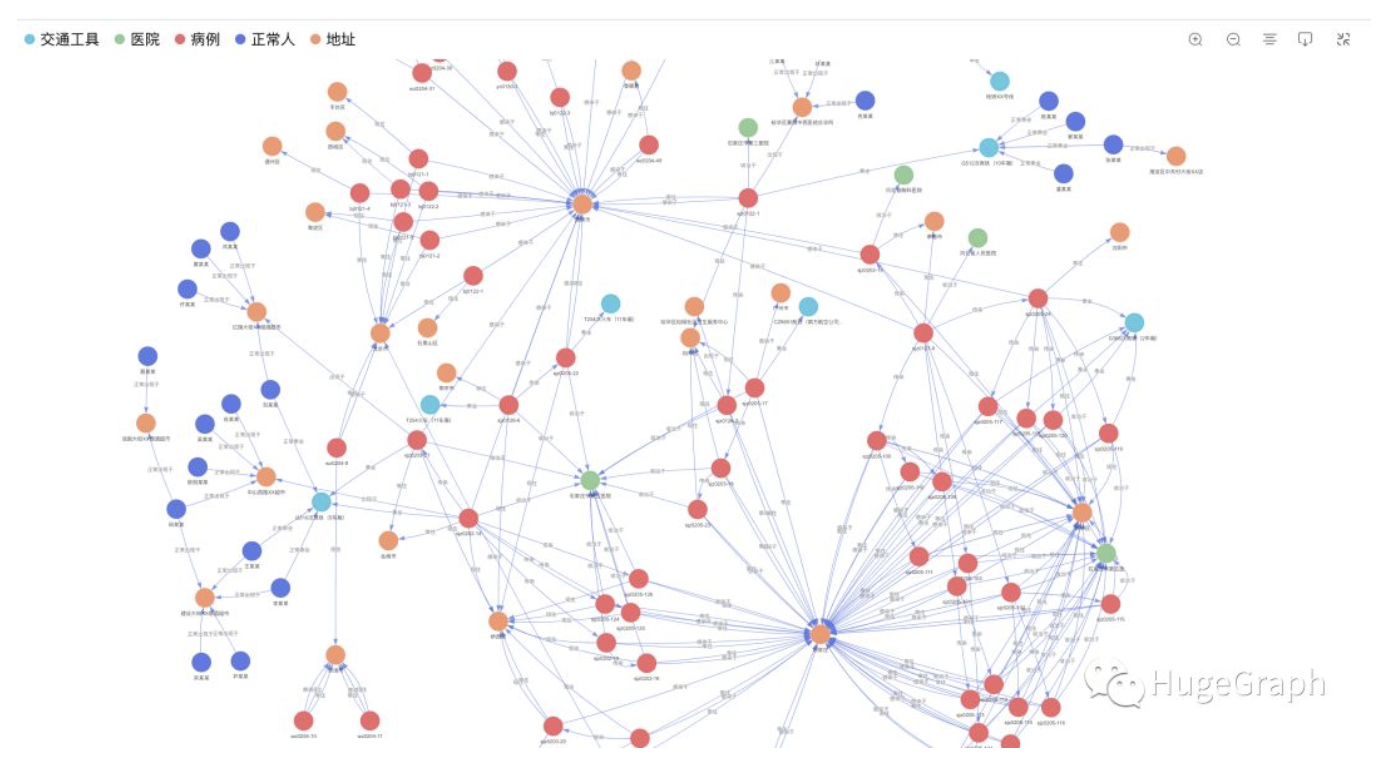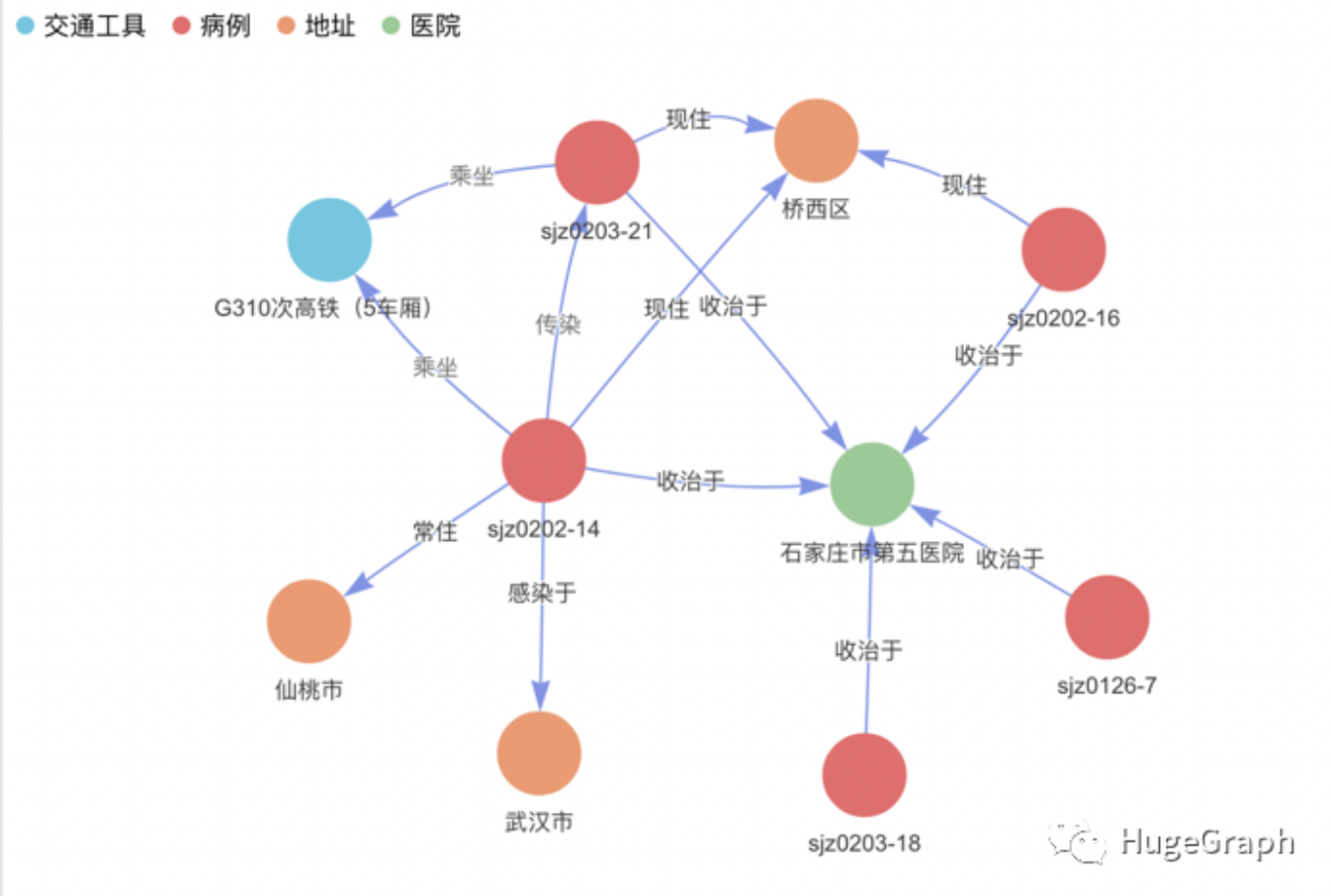1、病例数据：共导入了43条病例数据，包括病例的年龄、性别、感染原因、症状、确诊日期、省市等信息。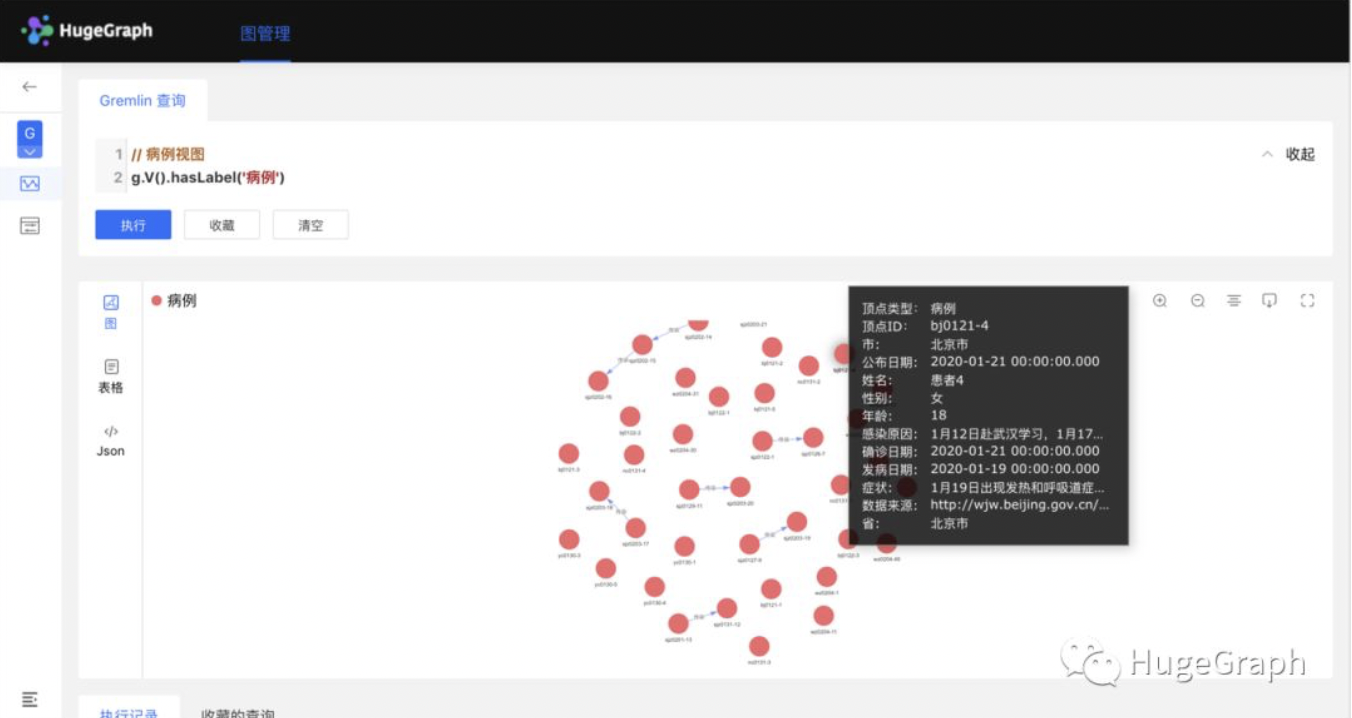2、地址数据：共导入了32条地址数据，比如“裕华区裕翔社区卫生服务中心”，主要包括上述病例出现过的地址。3、交通工具数据：共导入了6条交通工具数据，如高铁、航班、公交地铁等信息，主要包括上述病例乘坐过的交通工具。（这个数据较少省市公布）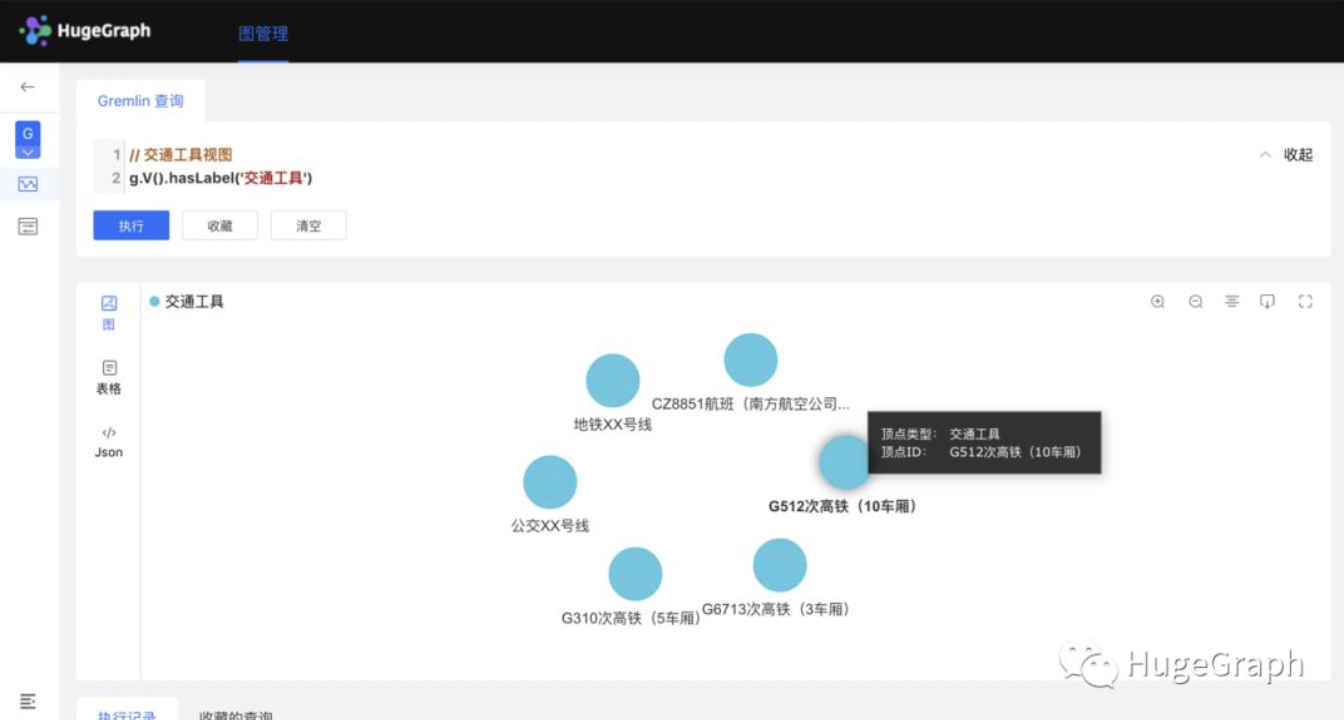4、医院数据：共导入了10条医院数据，如“北医科大学第四医院”，主要包括上述病例收治的医院。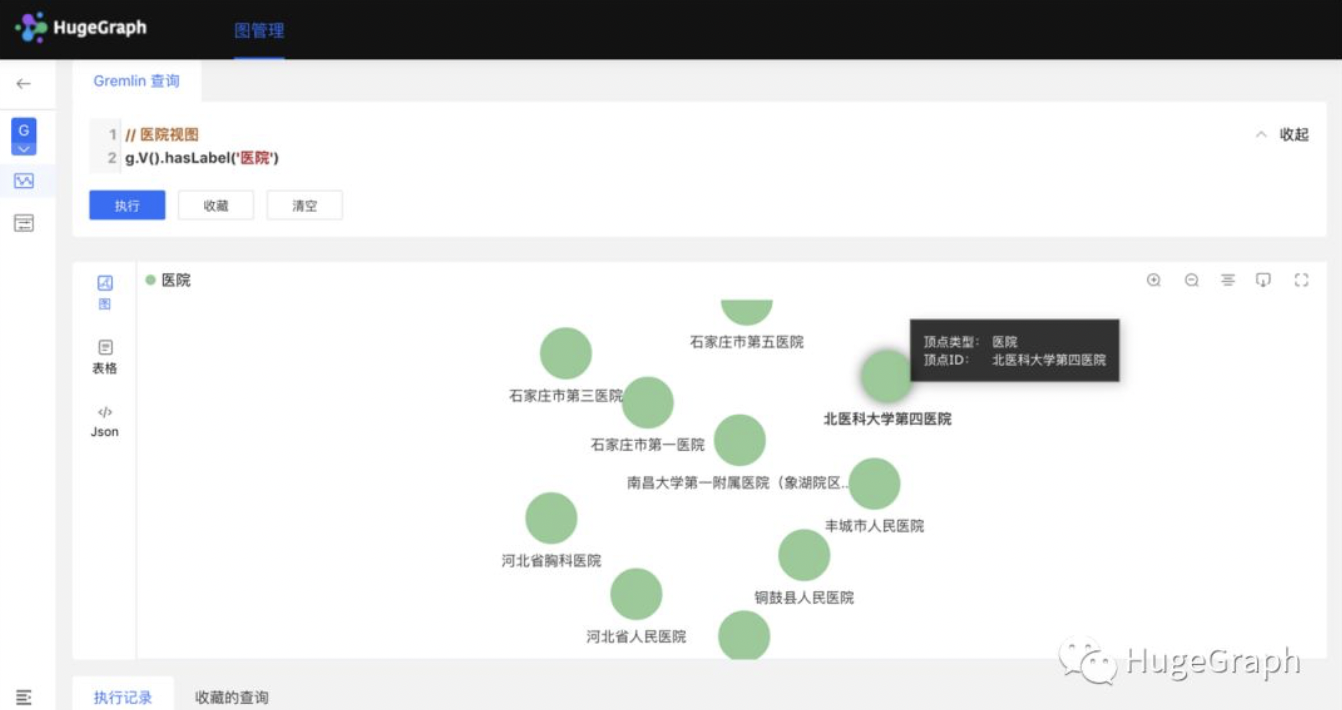5、“传染链”视图：共导入了8条传染数据，如“病例甲传染了病例乙”，主要包括上述病例和疑似病例的传染关系。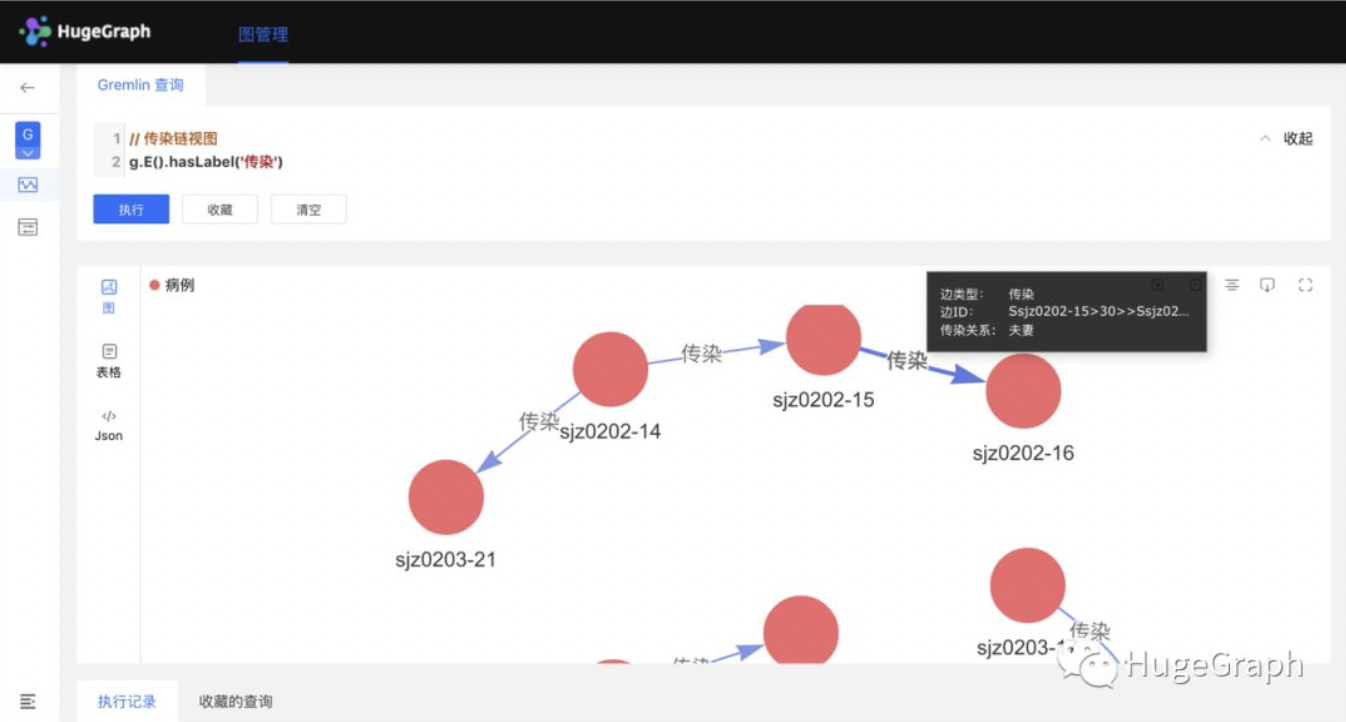6、“交通工具链”视图：共导入了8条交通工具乘坐信息数据，比如“病例甲在1月18日乘坐了G310次高铁”，主要包括上述病例和疑似病例的乘坐关系。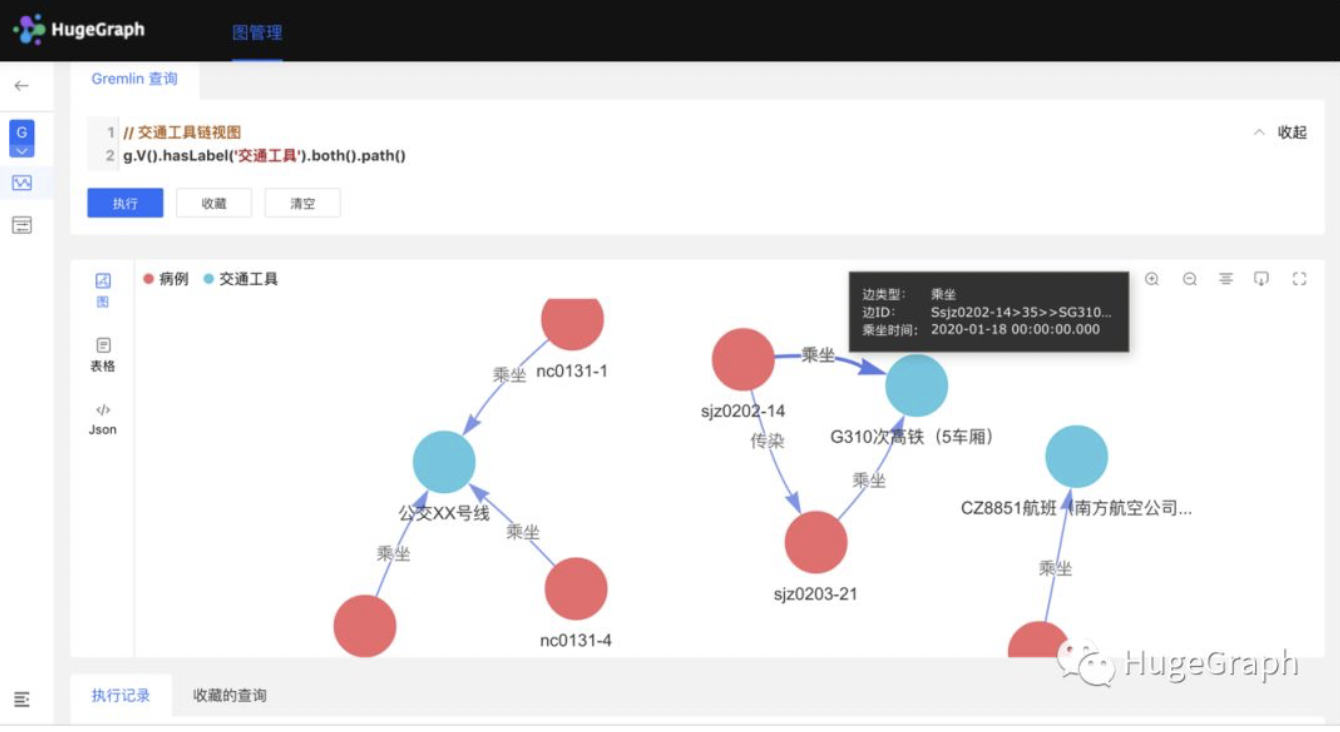7、“出现于场所”视图：共导入了9条病例、19条正常人出现的场所地址信息数据，比如“病例甲在1月21日10点到过XX超市”，主要包括上述病例和正常人的出现的地址关系。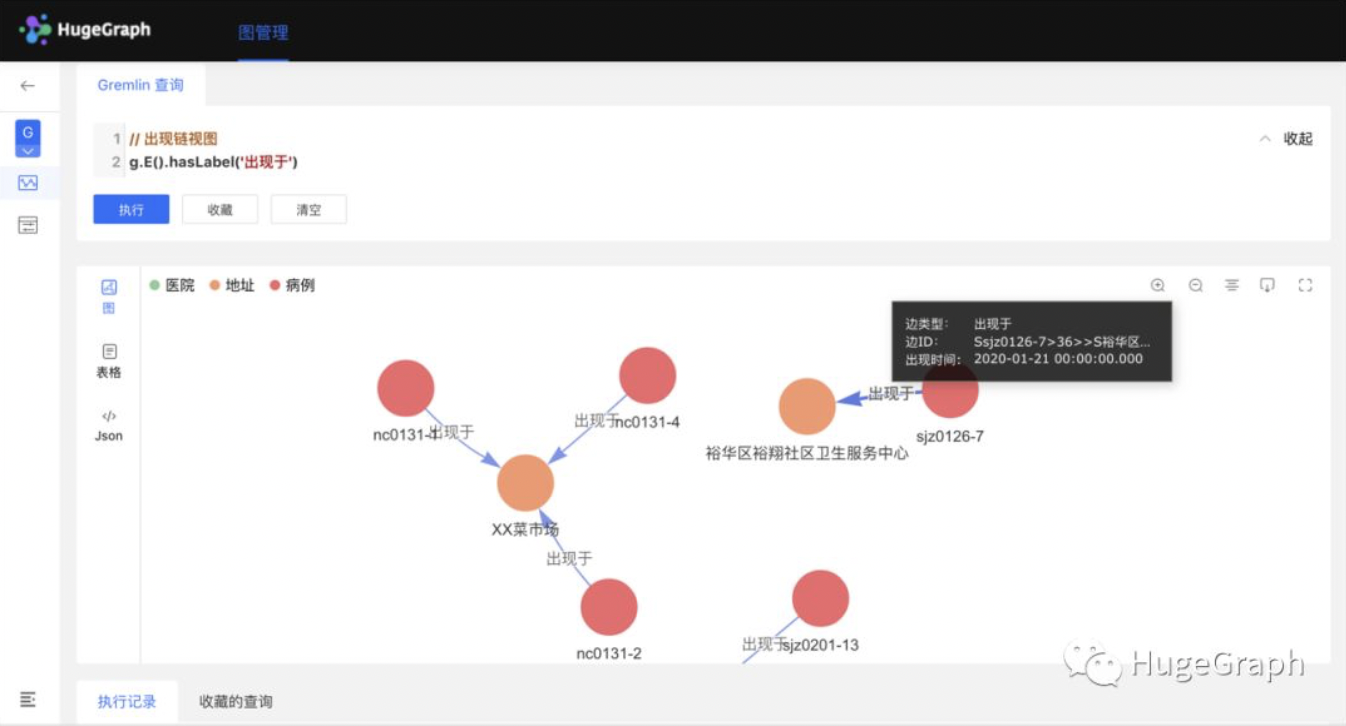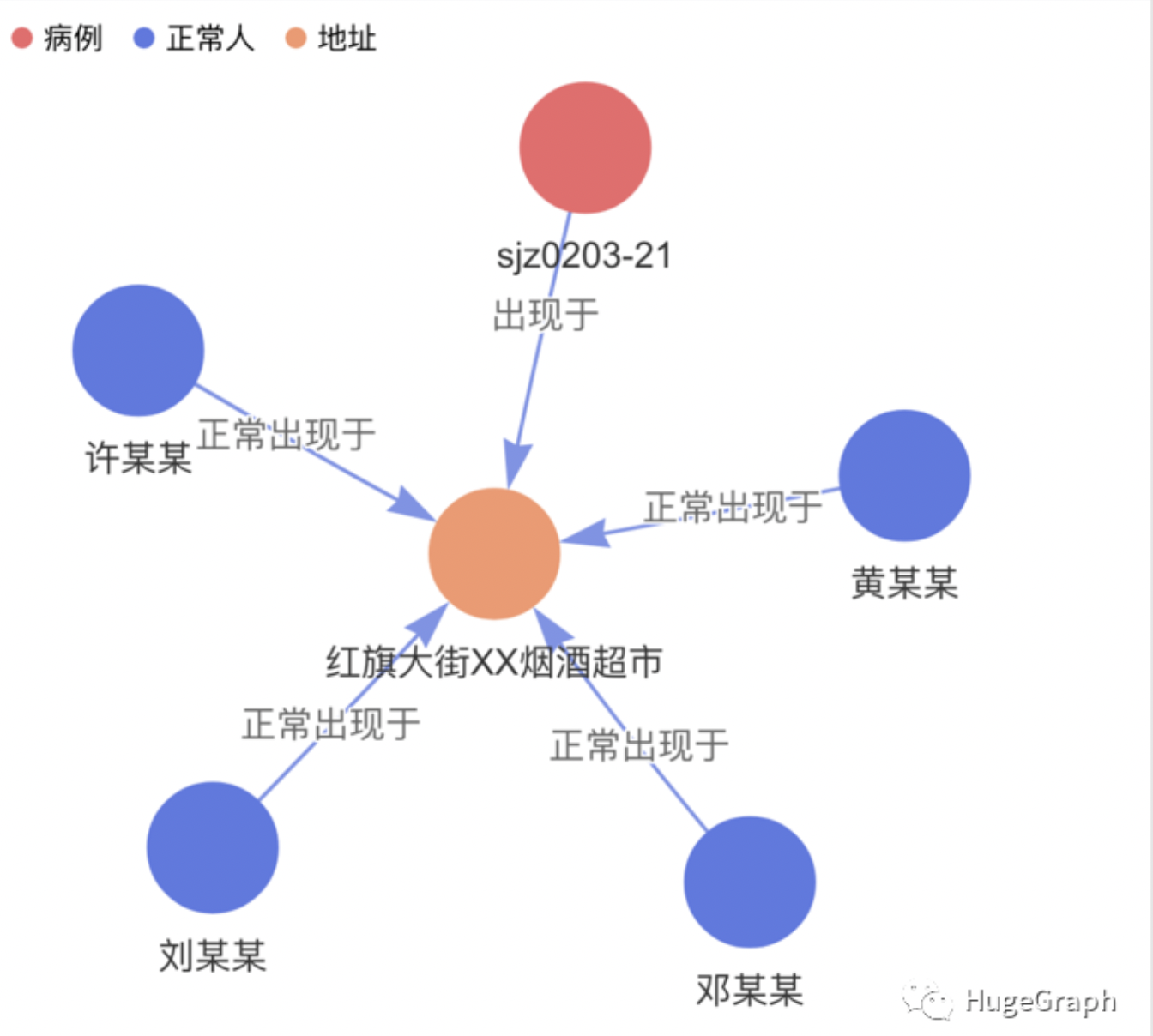8、某个病例关系链视图：除上述关联关系外，还导入了“感染于”、“现住”、“常住”、“收治于”等关系，主要包括上述病例和疑似病例的各种其它关系。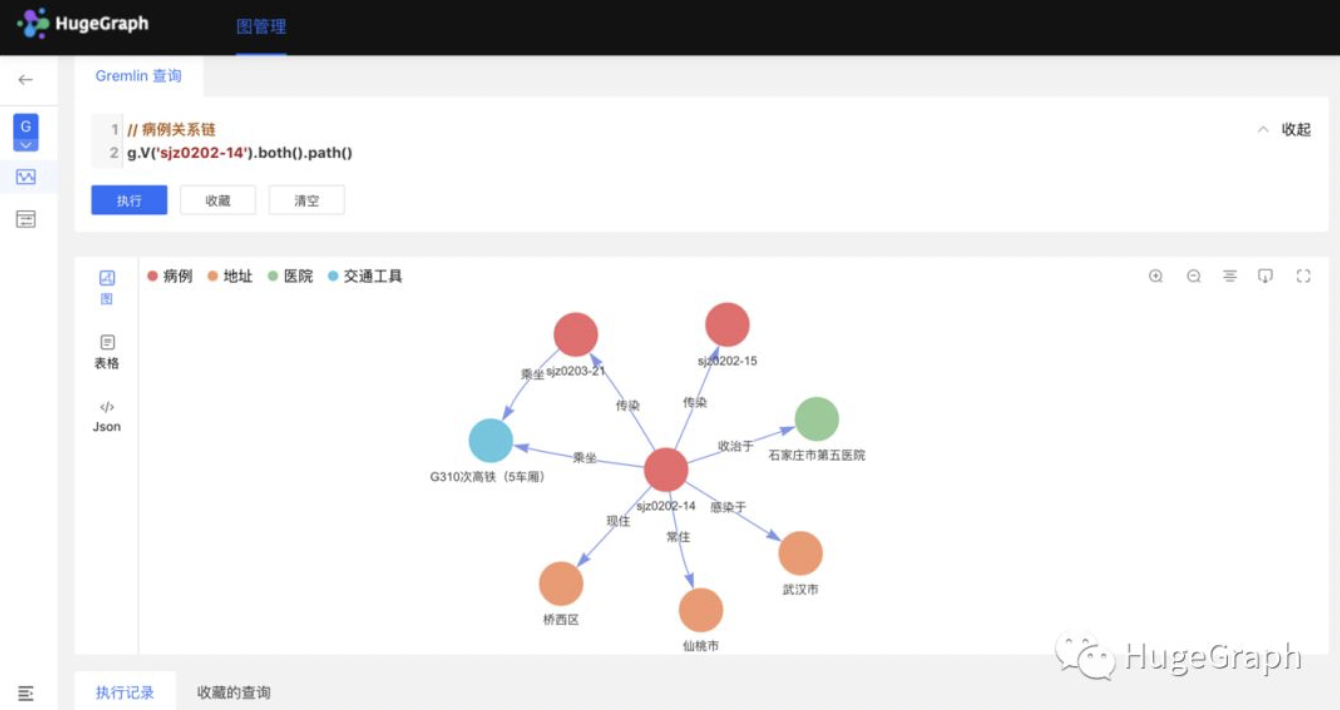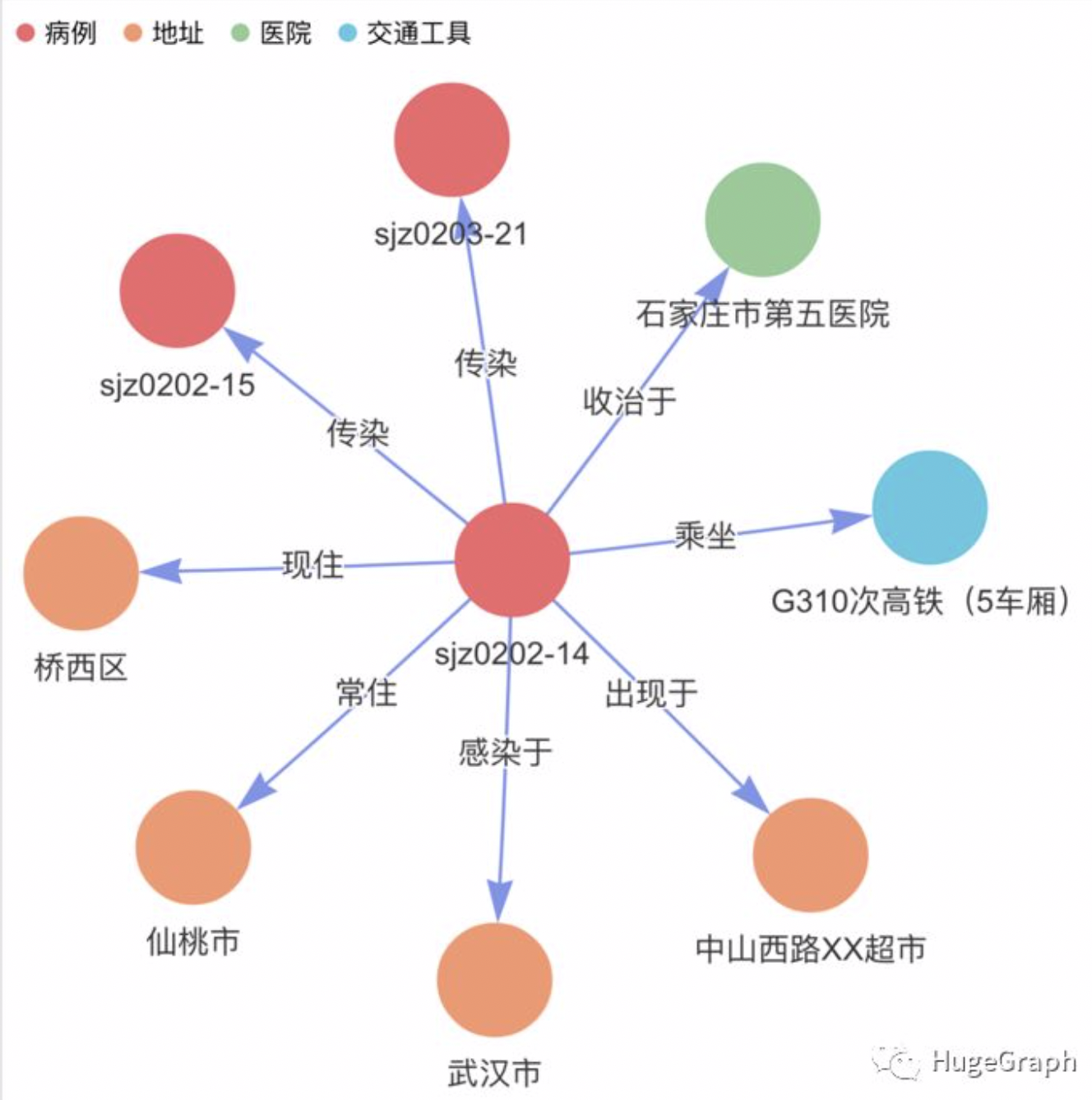9、“正常人”数据：共导入了22条正常人数据，包括姓名、年龄、性别、详细地址等信息；另外还导入了28条正常人的轨迹信息，如“某个正常人在1月19日乘坐过G512次高铁”、“某个正常人在1月20日10点到过XX超市”。(注意：正常人数据并不准确，仅供演示参考)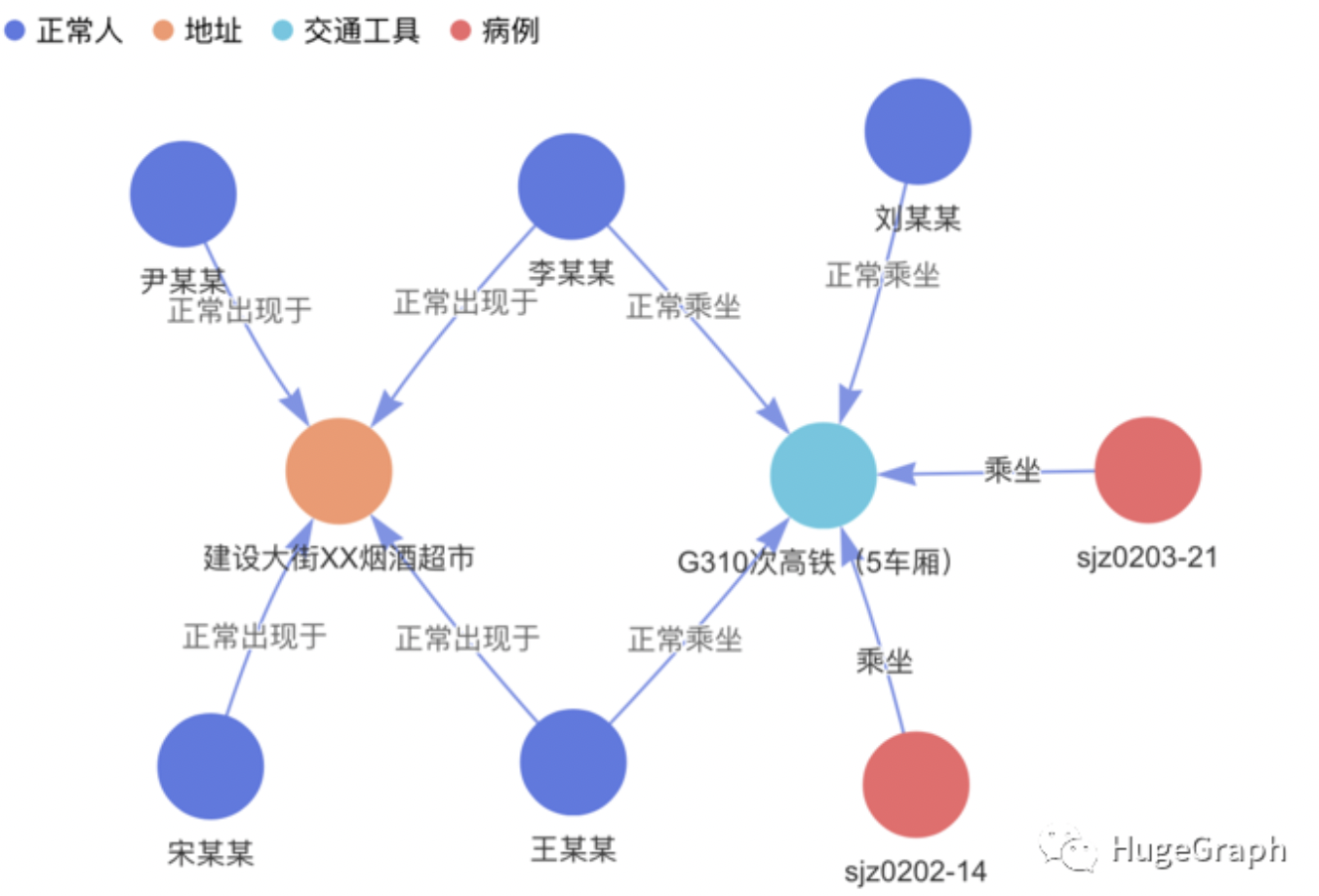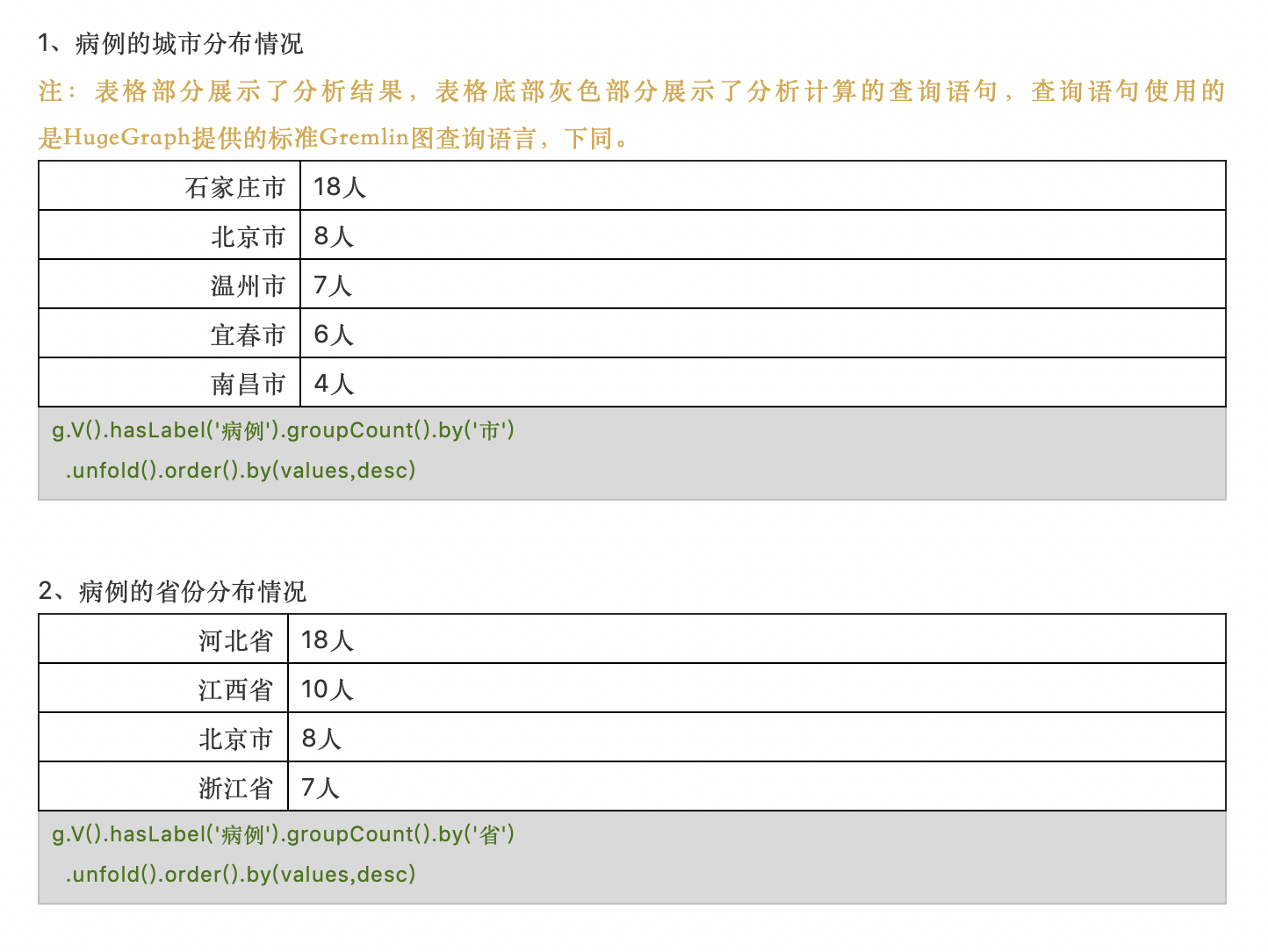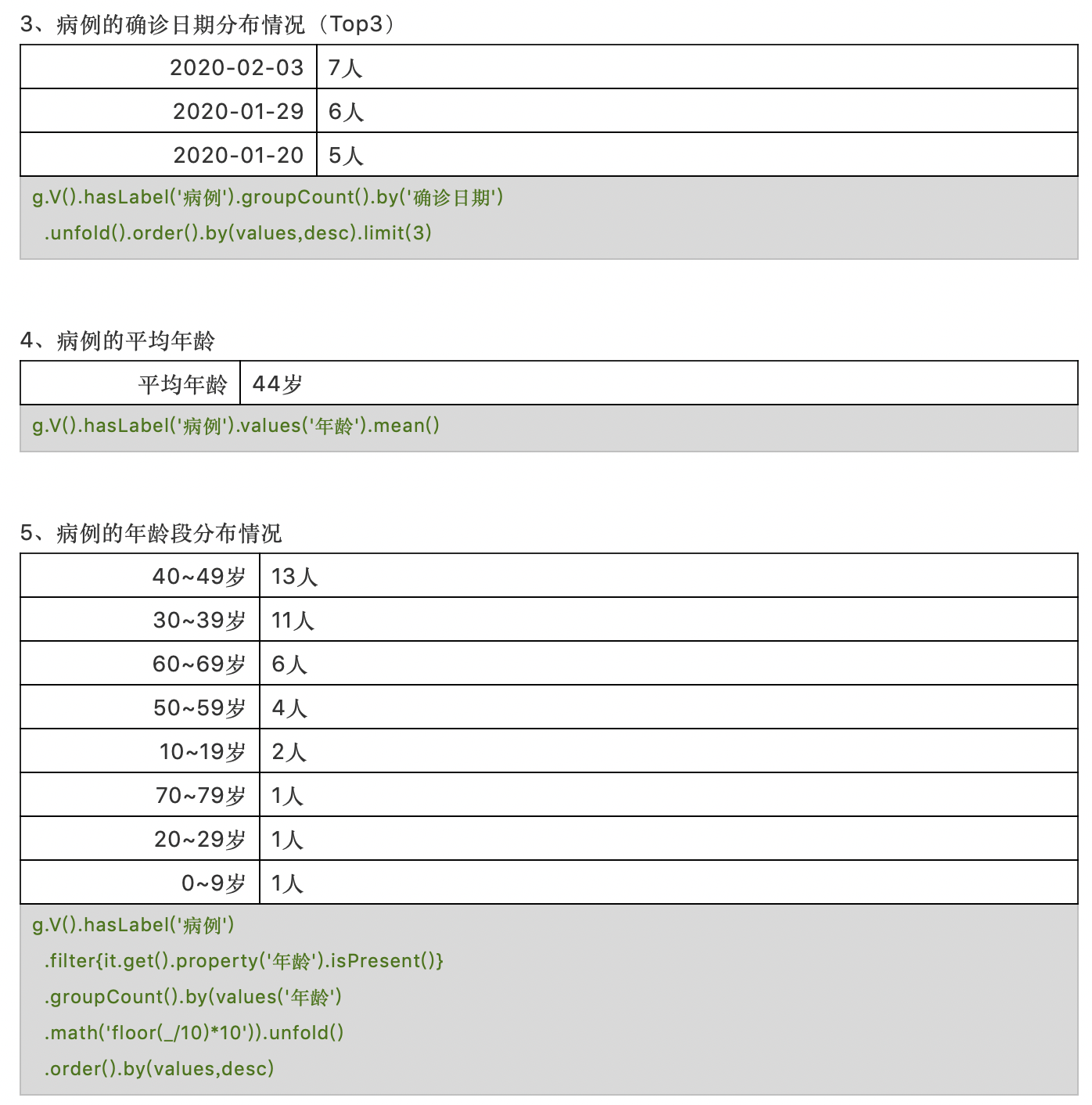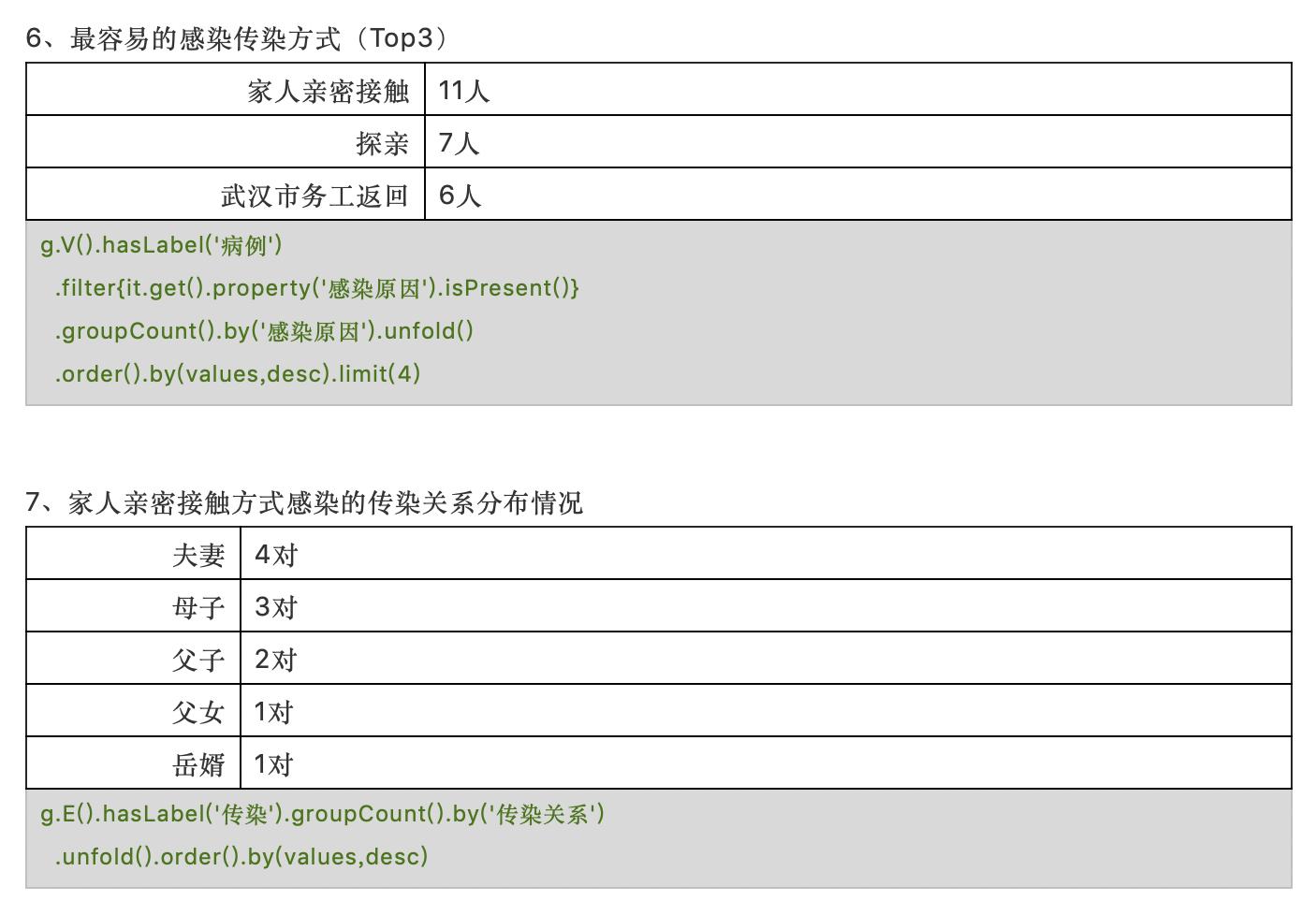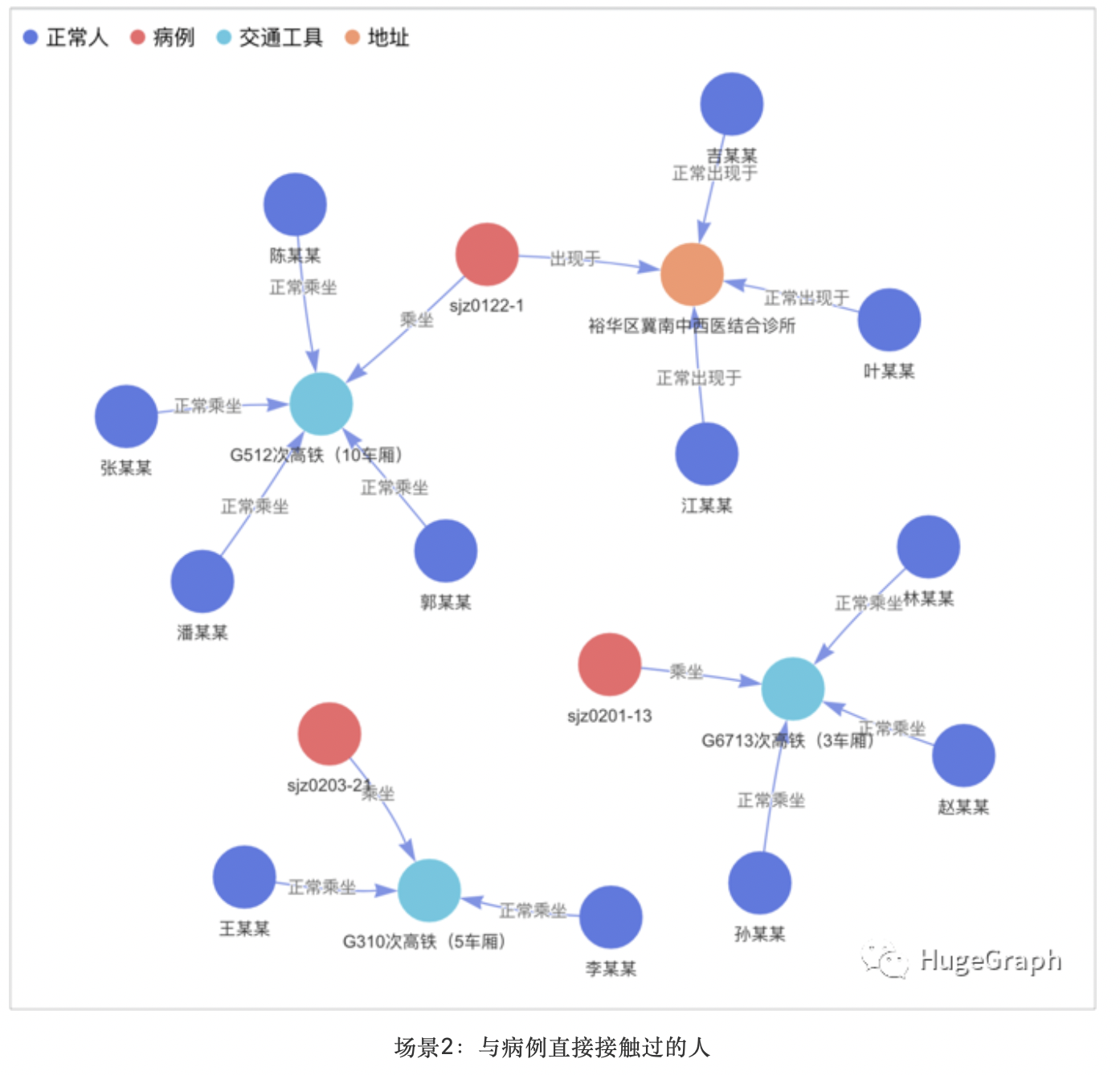``g.V().hasLabel('病例')`` .union(out('乘坐').in('正常乘坐').simplePath(),``        out('出现于').in('正常出现于').simplePath())`` .hasLabel('正常人').path()``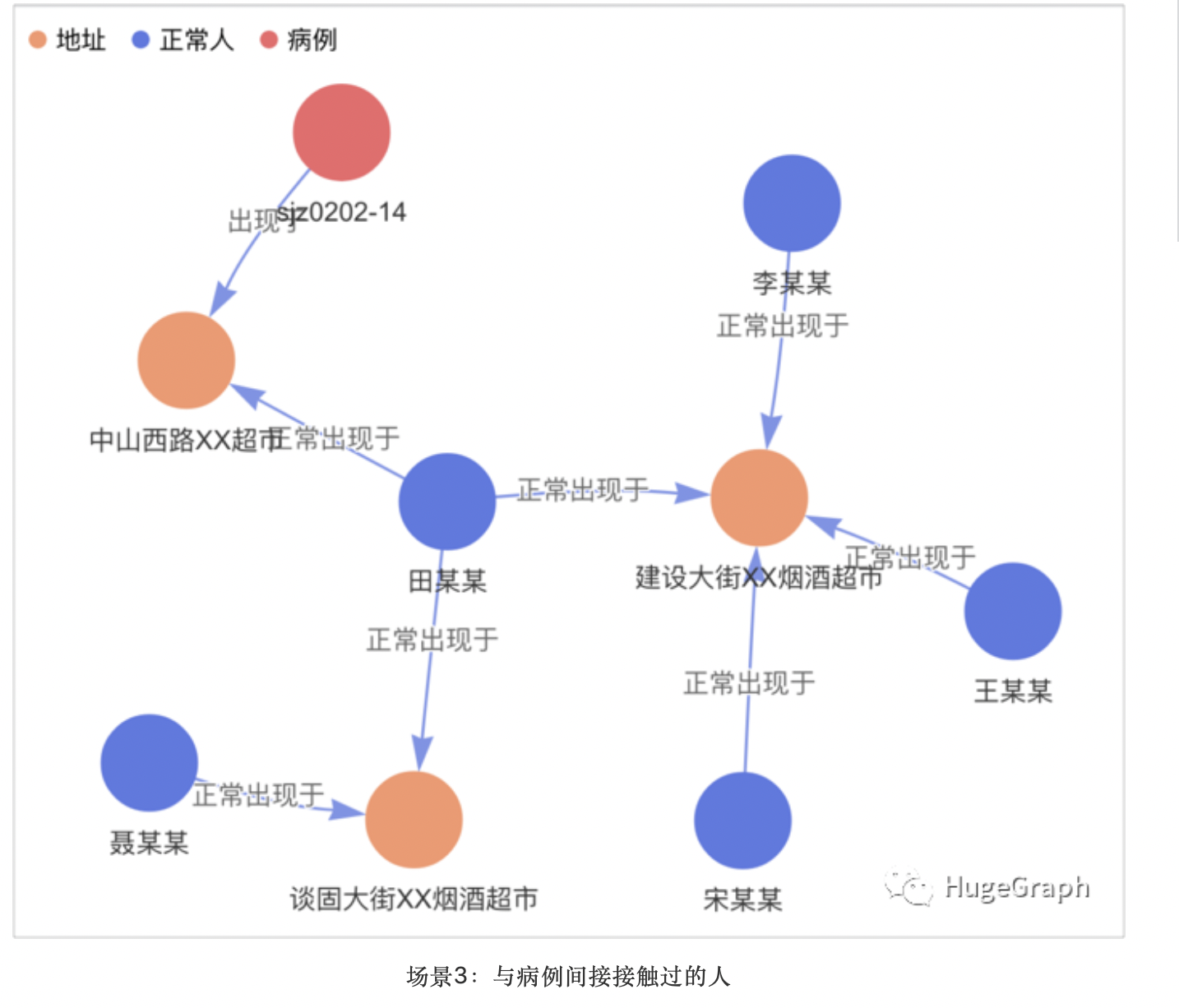``g.V().hasLabel('病例') .union(``  out('乘坐').in('正常乘坐').simplePath().out('正常乘坐').in('正常乘坐').simplePath(),``  out('出现于').in('正常出现于').simplePath().out('正常出现于').in('正常出现于').simplePath())`` .hasLabel('正常人').path()``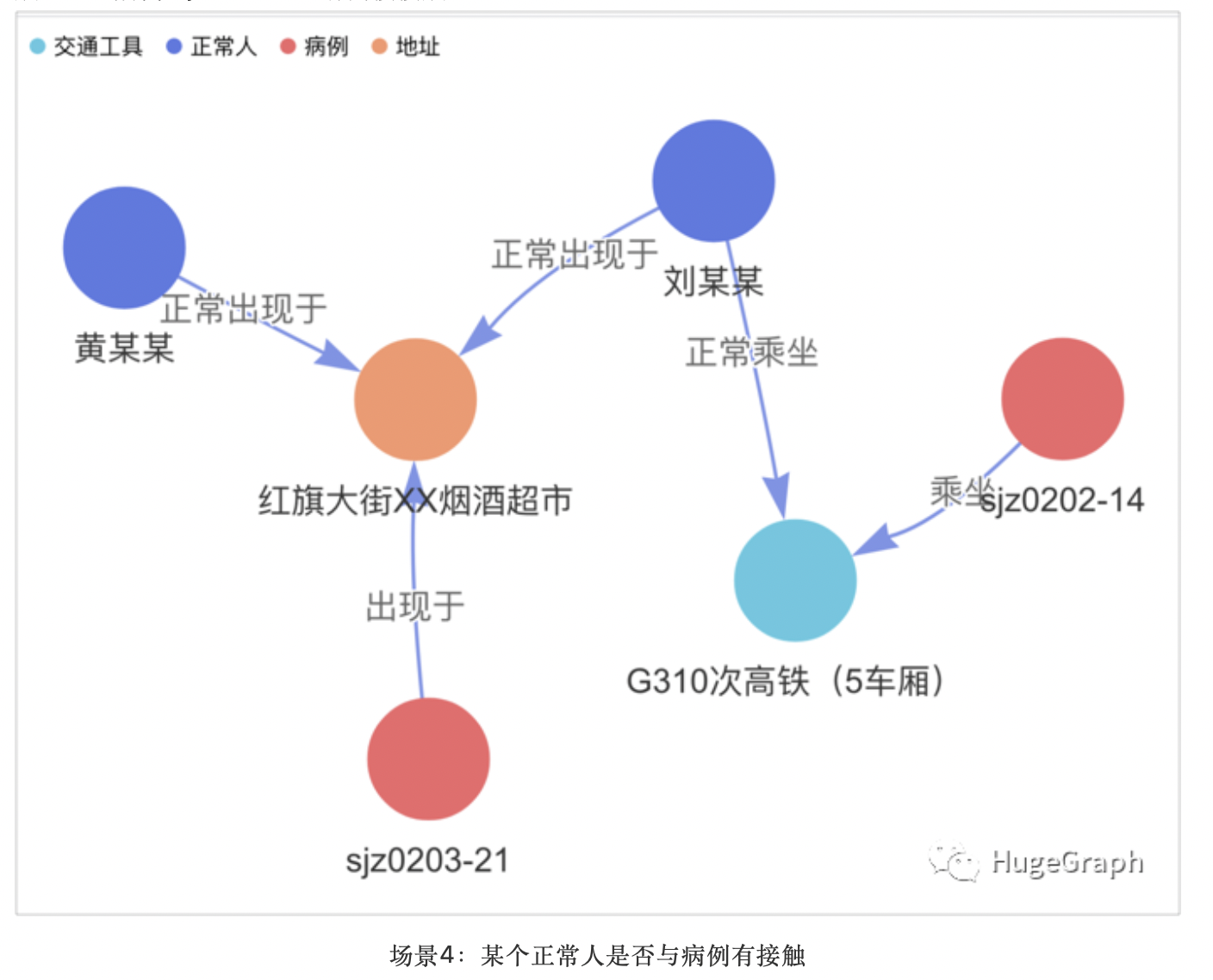``g.V('黄某某').repeat(out('正常乘坐','正常出现于')`` .in('正常乘坐','正常出现于','乘坐','出现于').simplePath())`` .times(2).emit(hasLabel('病例'))`` .hasLabel('病例').path()``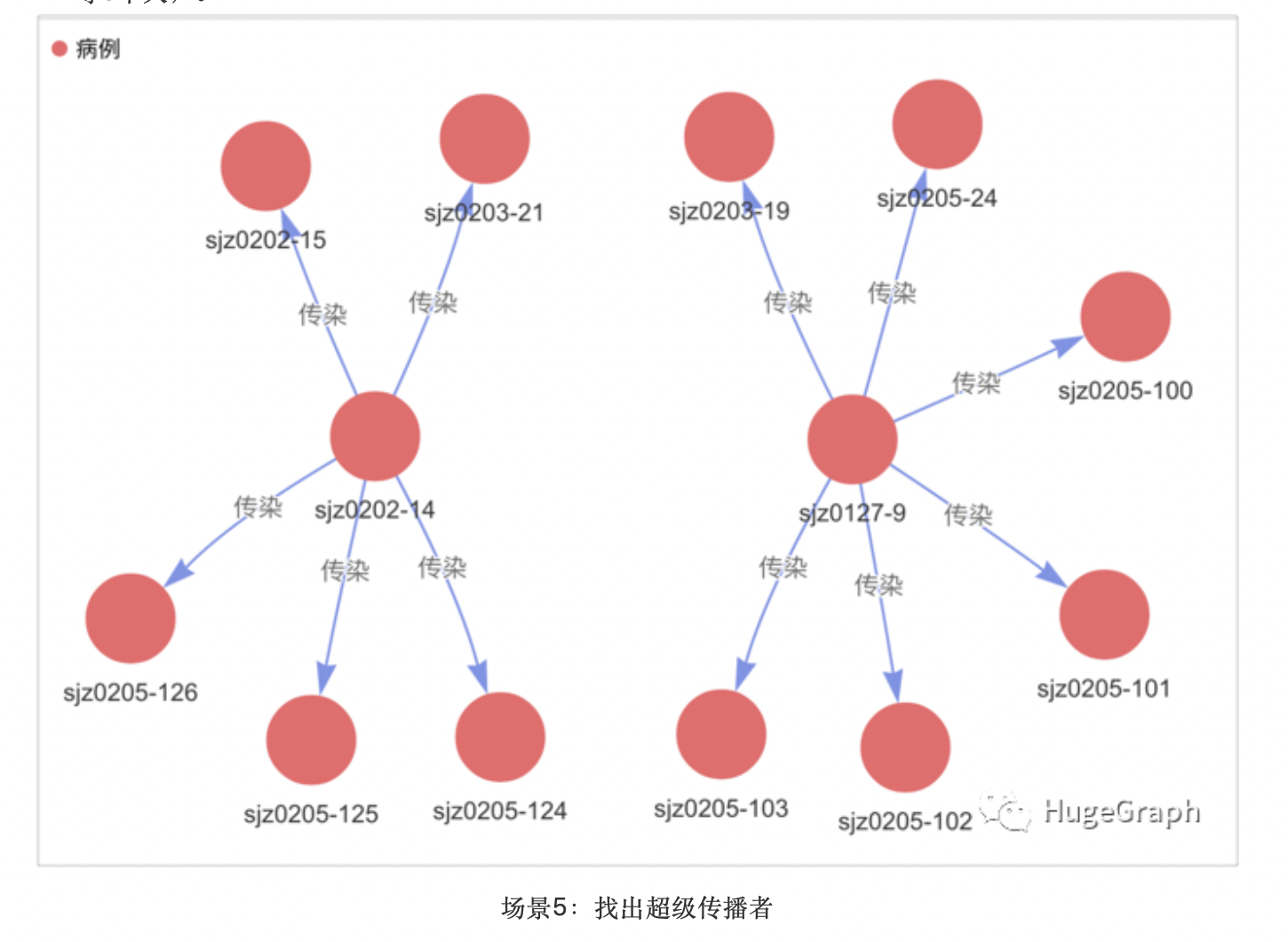``g.V().hasLabel('病例').where(outE('传染').count().is(gte(5)))`` .order().by(outE('传染').count(),desc).limit(10)``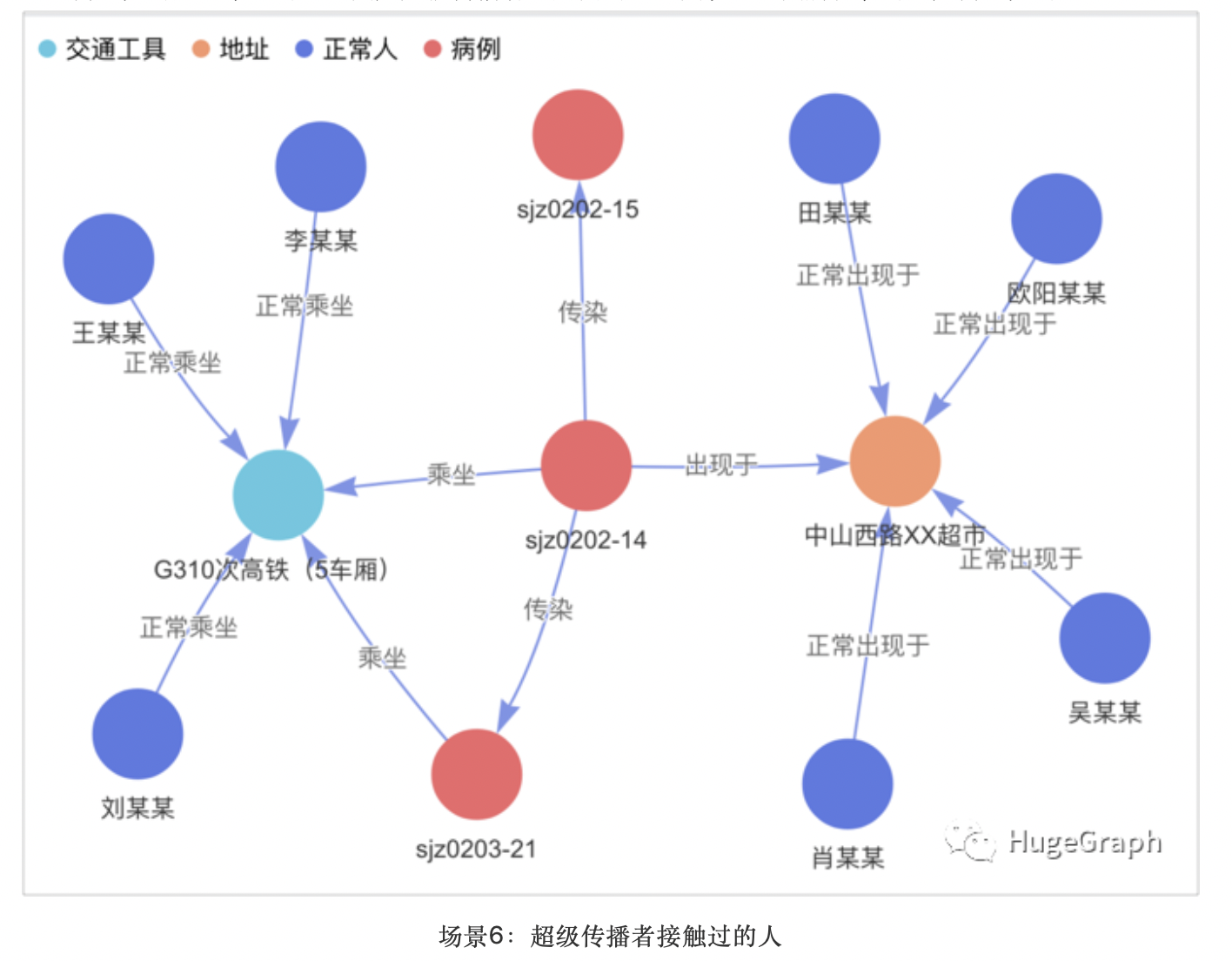``g.V().hasLabel('病例').where(outE('传染').count().is(gte(5)))`` .order().by(outE('传染').count(),desc).limit(10)`` .both().choose(hasLabel('病例'),identity(), both('乘坐','出现于','正常乘坐','正常出现于').simplePath())`` .path()``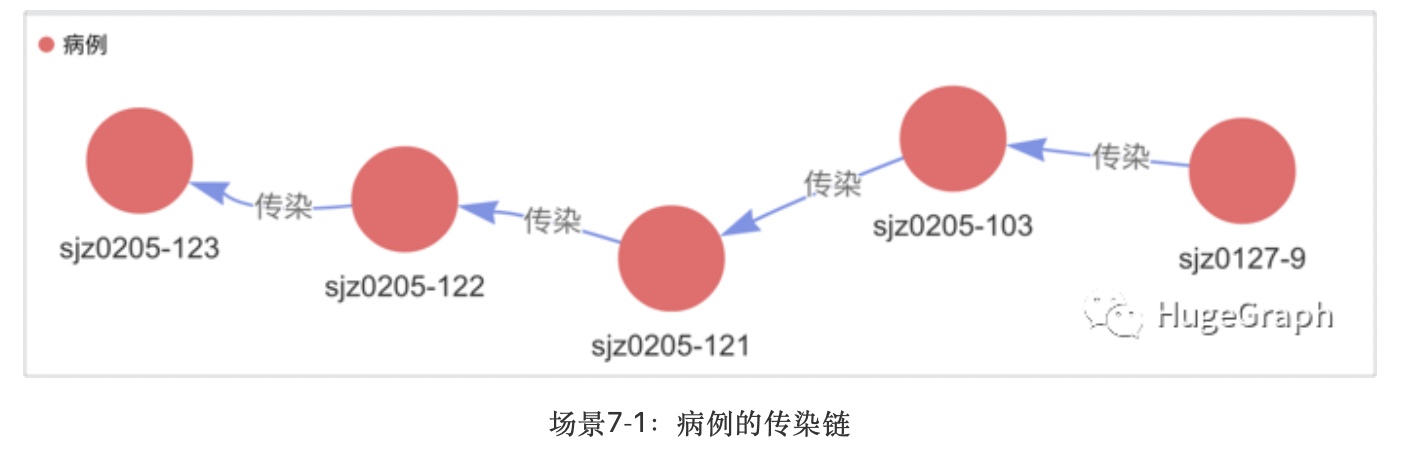``g.V('sjz0205-123').repeat(__.in('传染'))`` .until(__.not(__.in('传染'))).path()``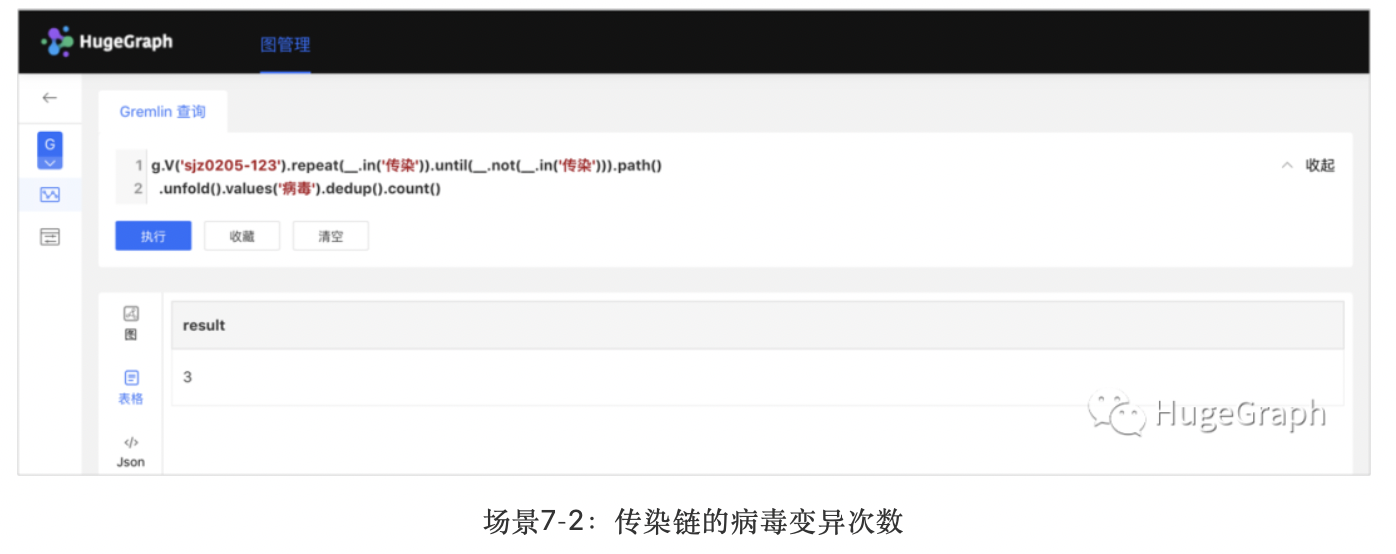``g.V('sjz0205-123').repeat(__.in('传染'))`` .until(__.not(__.in('传染'))).path()`` .unfold().values('病毒').dedup().count()``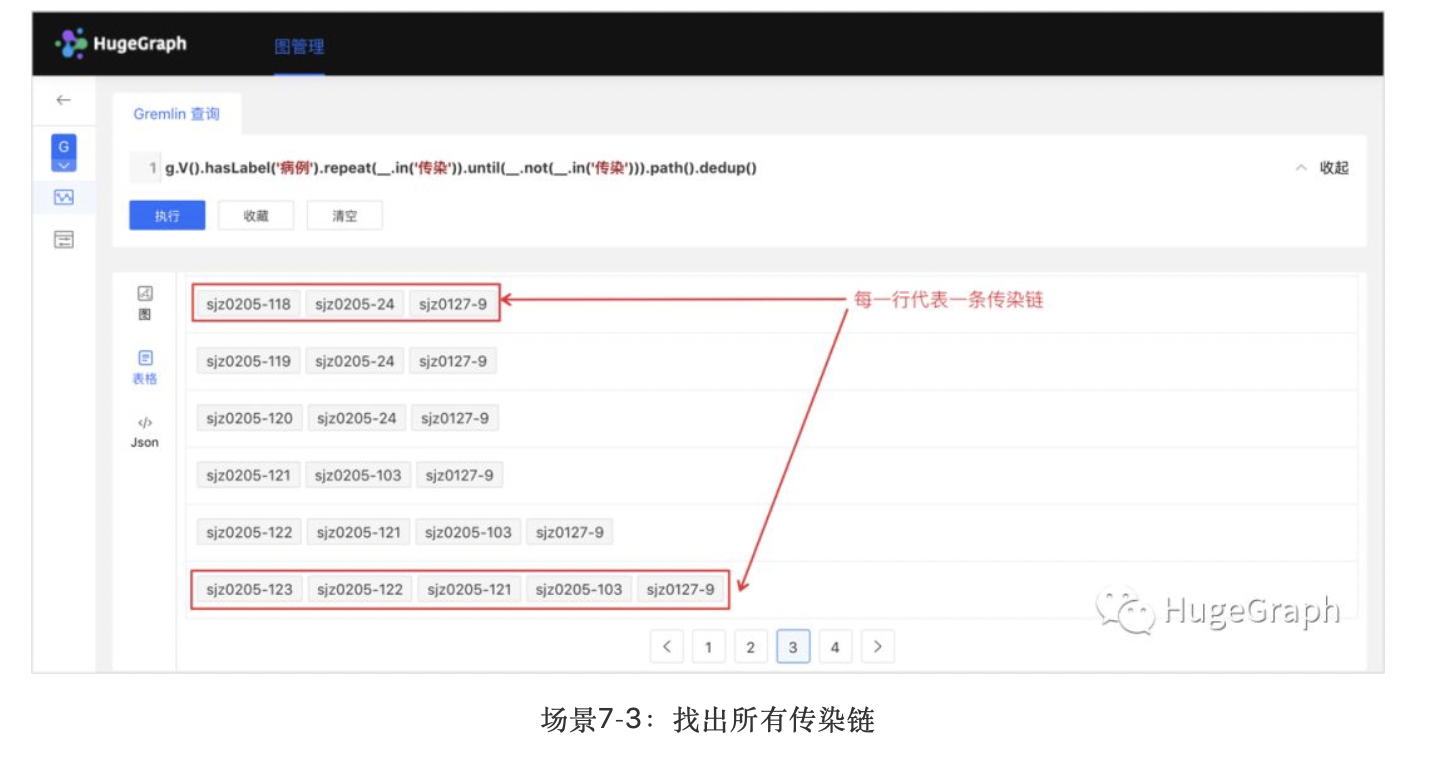``g.V().hasLabel('病例').repeat(__.in('传染'))`` .until(__.not(__.in('传染'))).path().dedup()``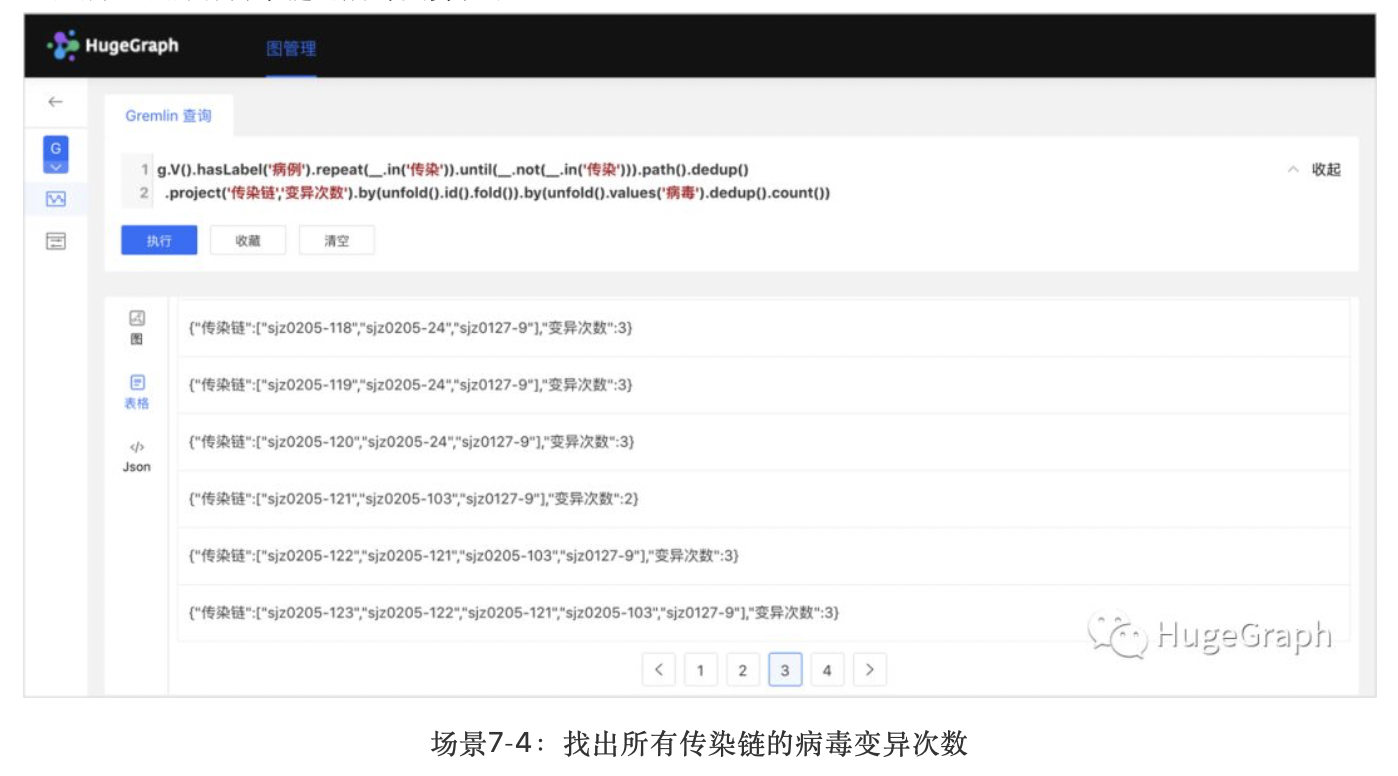``g.V().hasLabel('病例').repeat(__.in('传染')).until(__.not(__.in('传染'))).path().dedup()`` .project('传染链','变异次数').by(unfold().id().fold()).by(unfold().values('病毒').dedup().count())``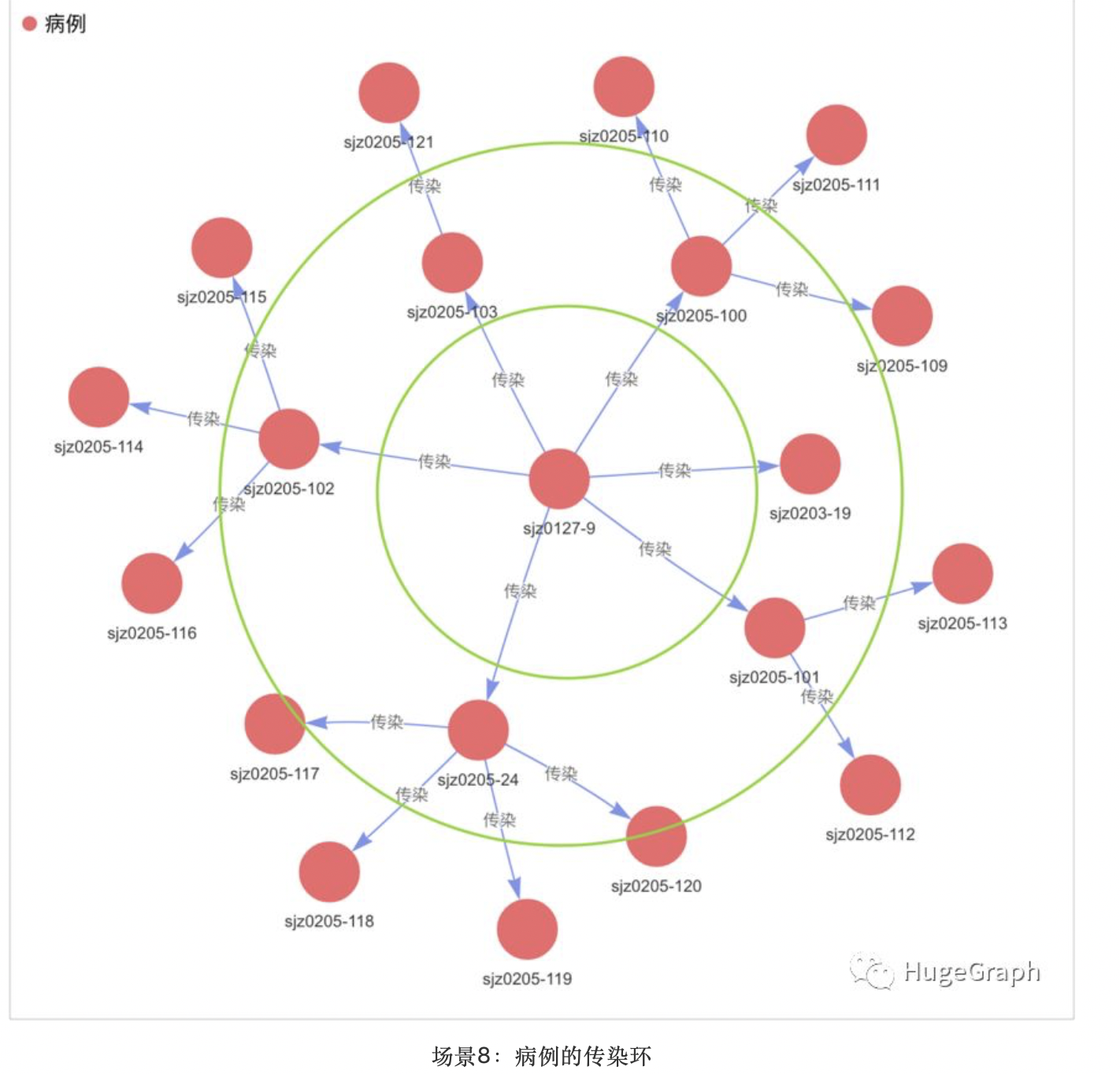g.V('sjz0127-9').repeat(out('传染')).times(2).emit().path()### HugeGraph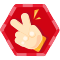### 评论(0)#### 暂无文章

【电薇同步;1.3.８ - ２.７.４.１ - 5.２.９.７.】张生、诚、信、合、作,保、真、售、后、保、障、长、期、有、效。adb的全称为Android Debug Bridge，是Android手机通用...

yyqqvip
21分钟前
30
0
Nginx 反向代理访问

25分钟前
33
0
python笔记：环境变量已设置CMD中一直报错"python"不是内部命令，也不是可运行的程序或批处理文件

27分钟前
13
0
AI+BI服务模式

zoegu228
28分钟前
20
0
leetcode1227(面试题 17.09. 第 k 个数)--C语言实现

55分钟前
27
0